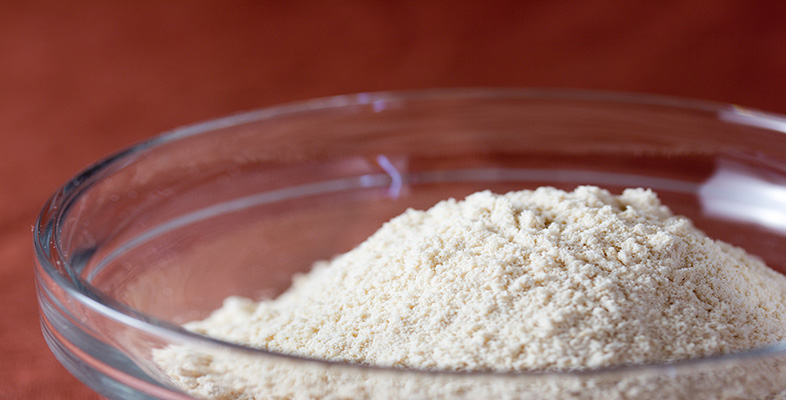Rounding and estimation

Start this free course now. Just create an account and sign in. Enrol and complete the course for a free statement of participation or digital badge if available.

Free course

# 1.6 Significant figures for numbers less than one

You can use the same procedure for numbers less than one.

## Example 4

In scientific work people deal with very small units of measurement. Suppose you read that the spacing between adjacent atoms in a solid was 0.000 002 456 84 metres. You could make the number more memorable by using two significant figure accuracy.

To find 0.000 002 456 84 metres correct to two significant figures, count from the first non-zero digit on the left. The first significant digit in this number is the 2. The zeros to the left of this digit are not significant but they maintain the place value of the other digits. The second significant digit is the 4 and to the right of this digit is a 5 which means round up to give 0.000 0025 metres (2 s.f.).

You may have noticed that the word ‘significant’ has a more precise meaning in mathematics than when used in everyday language and that sometimes zeros are not counted as significant figures, even though they are obviously important in specifying the place value of a digit.

At the beginning of Section 1.5 there was a £600 estimate for the cost of fencing. The zeros are necessary to indicate that the 6 represents six hundreds, rather than six tens or six units, but the fact that they are zeros (rather than another digit) is not significant (in the exact cost, it is unlikely that both would be zero). However if an exact calculation gives a cost of £609, then the zero is significant. (To tell you it is six hundred, zero tens and nine units.) This is a price quoted to three significant figures.

For decimal numbers like 0.060 09 metres, the zeros to the left of the 6 are not considered significant (although they are important to indicate the place value), but those to the right are significant.

## Activity 11

Round the numbers below:

• (a) to 1 s.f.

• (b) to 2 s.f.

• (c) to 3 s.f.

• (d) to 4 s.f.

0.042 6395,  0.000 289 99

• (a) 0.04,  0.000 3 to 1 s.f.

• (b) 0.043,  0.000 29 to 2 s.f.

• (c) 0.0426,  0.000 290 to 3 s.f.

• (d) 0.042 64,  0.000 2900 to 4 s.f.

## Activity 12

Round the numbers below:

• (a) to the nearest whole number

• (b) to 1 d.p.

• (c) to 1 s.f.

• (d) to 3 s.f.

238.483,  0.763 48,  0.088 8456

• (a) 238, 1,  0

• (b) 238.5,  0.8,  0.1

• (c) 200,  0.8,  0.09

• (d) 238  0.763,  0.0888

## Activity 13

On holiday it is often useful to do rough conversions into one's own currency to get a feel for how expensive an item is.

Suppose a Mexican Peso is £0.048 654. Convert this to (a) one significant figure, (b) two significant figures, and in each case estimate the cost, in pounds, of an item costing 278 Mexican Pesos.

• (a) £0.048 654 is £0.05, to 1 s.f.

So an item costing 278 Mexican Pesos will be about 278 × 0.05 = £13.90, or about £14.

If you rounded the cost to 1 s.f. too, you would have estimated 300 × 0.05 = £15.

• (b) £0.048 654 is £0.049, to 2 s.f.

So an item costing 278 Mexican Pesos will be about 278 × 0.049 = £13.62 (to 2 d.p.).

If you rounded the cost to 2 s.f. too, you would have estimated 280 × 0.049 = £13.72.

MU120_4M2